### Energy

##### 1. Convergence Test: Cutoff Energy
2019-07-24 13:11:40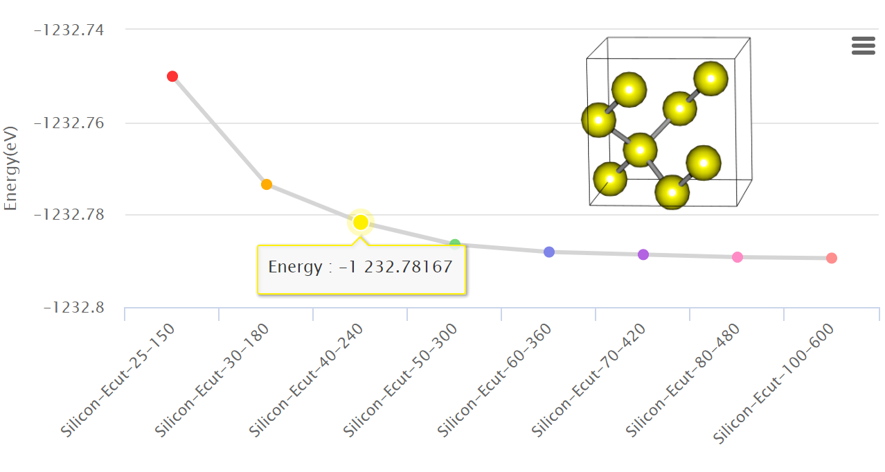Convergence test is a way of optimizing a simulation to use limited computational resources efficiently. It is important to find a proper initial input setting for simulation research to decide the accuracy and reliability of the simulation.

##### 2. Convergence Test: K-points
2019-07-24 13:16:02In the first email that we sent last week, we briefly introduced convergence tests and how to conduct one by using the total energy. This time, we would like to explain how to perform the test by using the number of k-points.

##### 3. How to Calculate Cohesive Energy
2019-07-24 13:20:38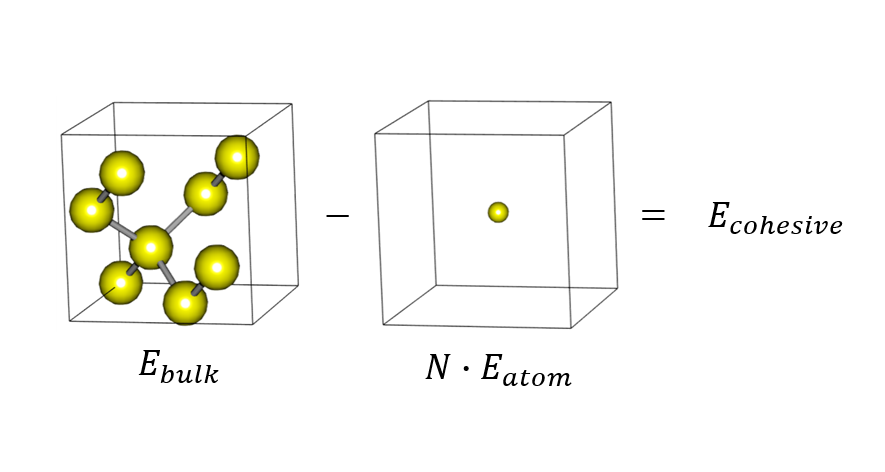The energy for bonding can be calculated using the difference between a bonded structure and a dissociated one. This energy, which is necessary to separate an atom from the solid, is called cohesive energy.

##### 5. Calculation of a Single Atom
2019-07-24 13:41:58Cohesive energy, which is defined as the difference between the energy per atom in a solid and the energy of a single atom, is needed when an atom is separated from the other atoms comprising a material. It can be used to understand the property of crystal such as in finding the correlation effect of an ionic crystal or identifying the stability of the surface.

##### 10-1. Adsorption Energy Obtained through Slab Structure
2019-07-25 01:46:45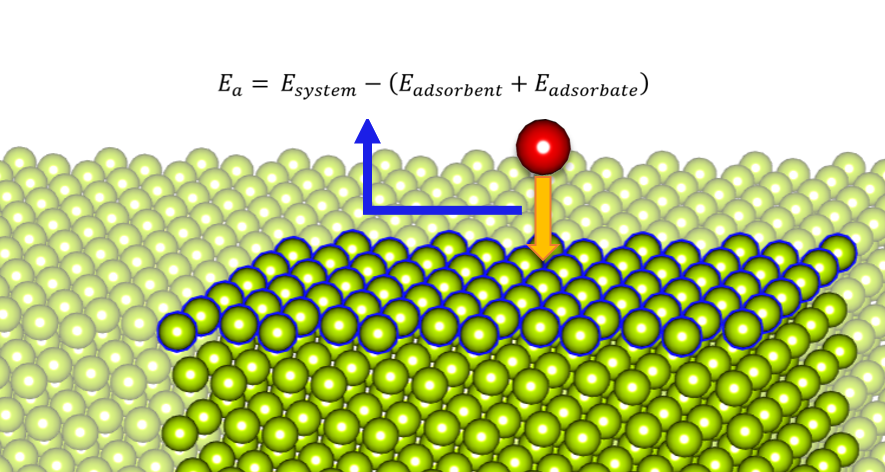Under the adsorption process in which an atom, ion, or molecule (adsorbate) is attached to the surface of a solid (adsorbent), the energy that decreases while two materials are combined is called adsorption energy. This energy is mainly used when calculating the chemical engineering properties, exploring the adsorption mechanism, or determining the energetic heterogeneity of the surface of a solid.

##### 10-2. Surface Energy Obtained through Slab Structure
2019-07-25 02:01:51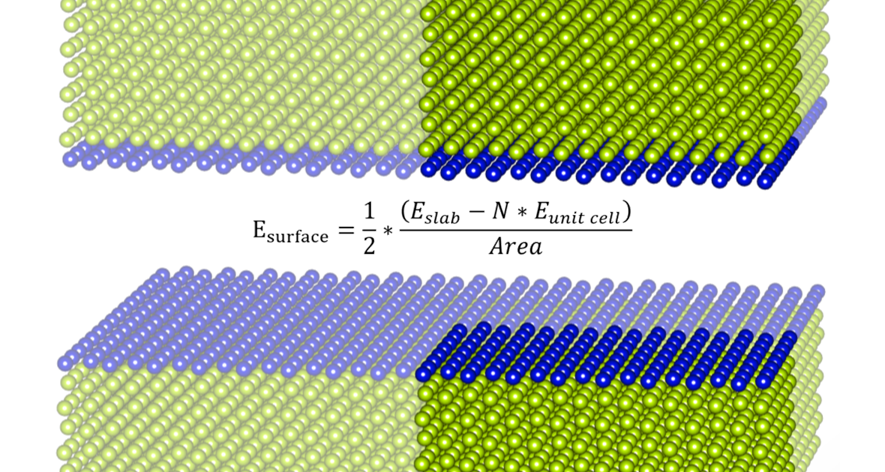When modeling a slab structure, after the relaxation of the unit cell, use the optimized unit cell to create a slab structure. Then, it can reduce the time for calculating a slab structure. In addition, with the use of the two energies, you can get the surface energy.

##### 11. Stacking Fault Energy
2019-09-09 06:00:11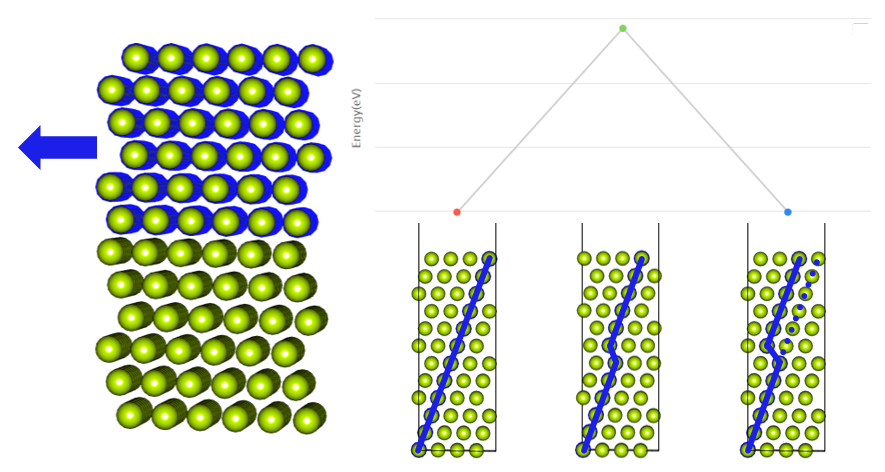If some of the crystal is moved, a stacking fault is created. The energy generated by the stacking fault is called stacking fault energy (SFE). The stacking fault energy can be used to determine the thermodynamic stability of a crystal structure or to find out the deformation behavior.

##### 16. Bulk modulus
2019-09-09 06:25:11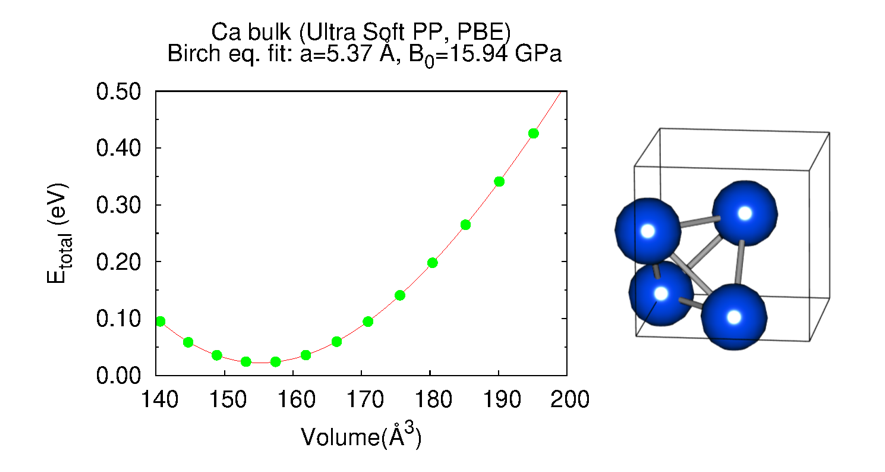Assuming that a material is isotropically compressed by external pressure (ΔP), its volume will change from V to V+ΔV. At a small volume change, ΔP is proportional to the volume strain ΔV/V, and the proportional constant (K) is called the bulk modulus. Can calculate the bulk modulus by applying the data obtained from the several executions of scf.

##### 19. Cohesive Energy with Different Pseudopotentials
2019-08-21 09:36:06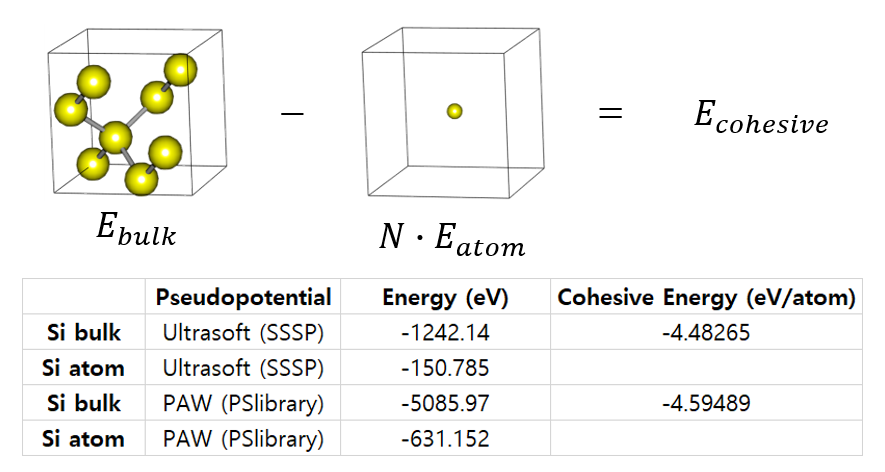Different pseudopotentials yield different energy values. Therefore, energy values ​​should not be compared directly. But the trend is consistent. So compare the difference in the values.

##### 22. Formation Energy
2019-09-11 04:39:52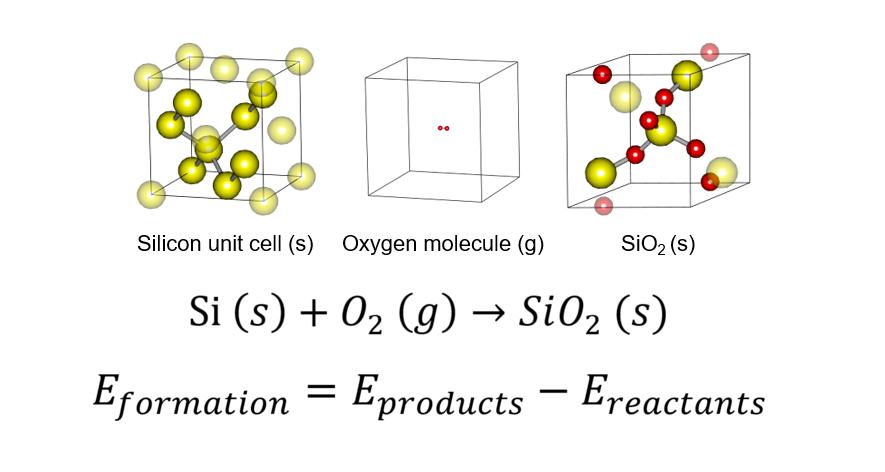Formation Energy is the value to be used as a measure of the amount of energy entering and exiting a chemical reaction, thus providing a basis for determining thermodynamic stability.

### DOS

##### 6. Easy to Get, but Contains a Lot of Information, Density of States(1)
2019-07-24 13:51:44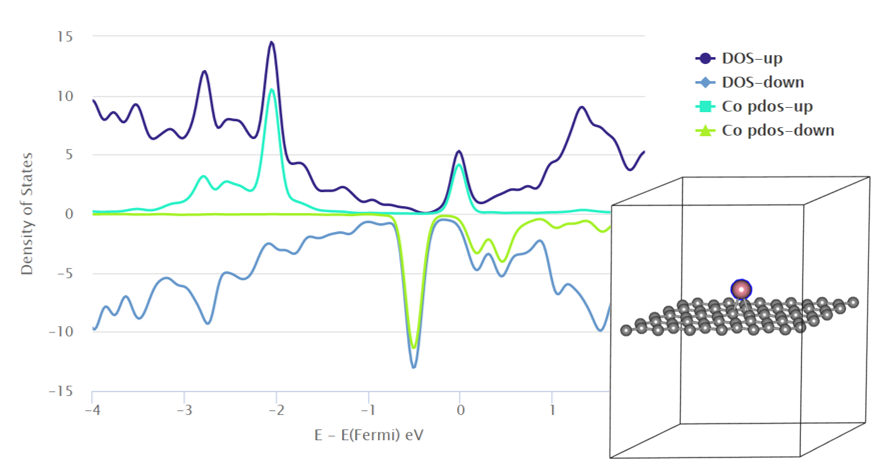The density of states (DOS) is the number of different electron states whose occupation is allowed in a specific energy level, which is (N(states)∙E-1V-1). DOS is a very important concept to describe the properties of a macroscopic system quantum-mechanically. Moreover, it is one of the calculation data frequently used when obtaining the electronic structure of a substance. In terms of an experiment, it can be measured by the photoemission spectroscopy (PES), which is a photoelectron experiment. In addition, in terms of computer simulation, it is usually calculated by using the Kohn–Sham equation.

##### 8. Easy to Get, but Contains a Lot of Information, Density of States(3)
2019-07-24 14:43:52Quantum Espresso provides “projwfc.x,” a postprocessing code for calculation of DOS. Projwfc.x is used to calculate the projection of the wave function of an atomic orbital. The projected DOS data obtains a graph by plotting the energy (E – EF) that Fermi energy is corrected. As DOS is calculated with the basic energy calculation (pw.x) in Materials Square, the calculation of DFT in the Quantum espresso module can easily give DOS data.

### Charge Density

##### 17. Charge Density Difference
2019-09-18 09:14:22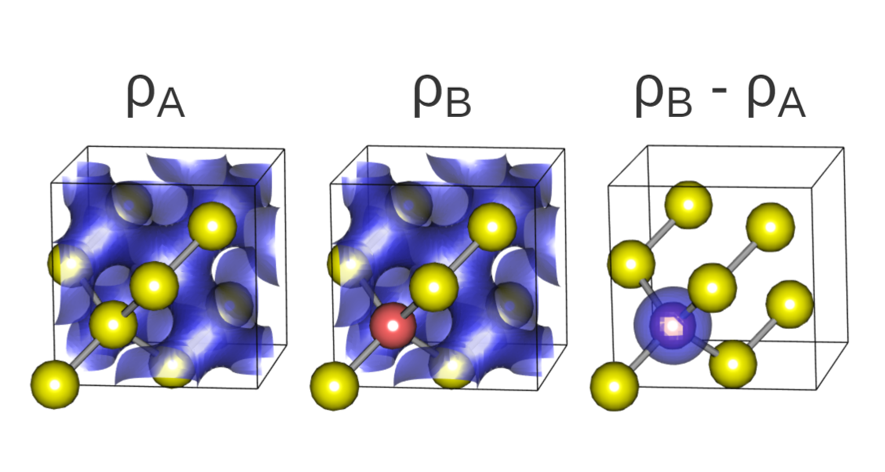With a DFT calculation, you can calculate an electron charge density. With Materials Square, you can conveniently find and visualize the charge density data by carrying out a plane-wave basis DFT calculation of Quantum Espresso.

##### 20. Dipole Correction
2019-08-29 07:20:16The artificial electric field added automatically to delete a 'potential jump' in the boundary to asymmetry slab structure. So we should do dipole correction by adding a 'sawtooth potential' to the vacuum region to delete the artificial electric field.

### Band Structure

##### 12-1. Band structure (Insulator, Metal)
2019-09-09 06:05:11A hexagonal diamond is considered as an insulator because it has a sufficient bandgap and the Fermi level is observed within the bandgap. On the other hand, the band structure of aluminum is a conductor because the conduction and valence bands are overlapped without any gaps near the Fermi level.

##### 12-2. Band structure (Silicon)
2019-09-09 06:09:11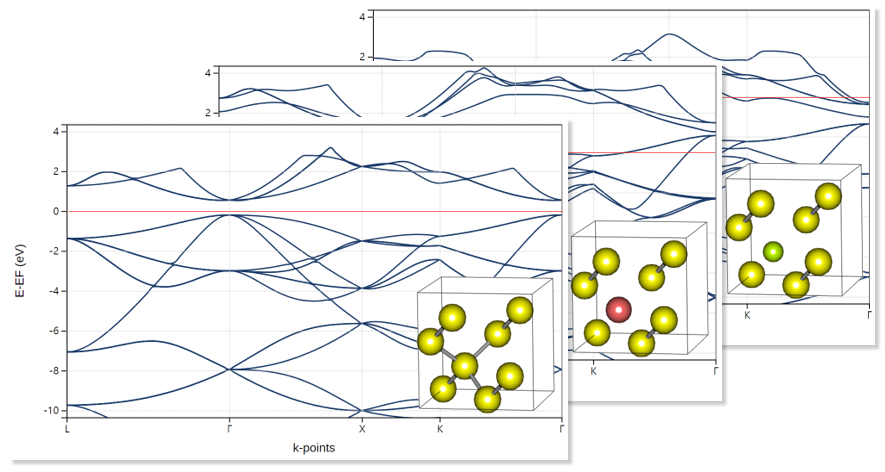When you observe the band structure of silicon, the band gaps and the Fermi level existing between them can be seen. However, if P is doped, the band gap moves below the Fermi level, thus causing it to be conductive. On the other hand, if B is doped, the band gap moves above the Fermi level, thus causing it to be conductive as well.

##### 13. Band Structure (k-point, nscf)
2019-09-09 06:19:11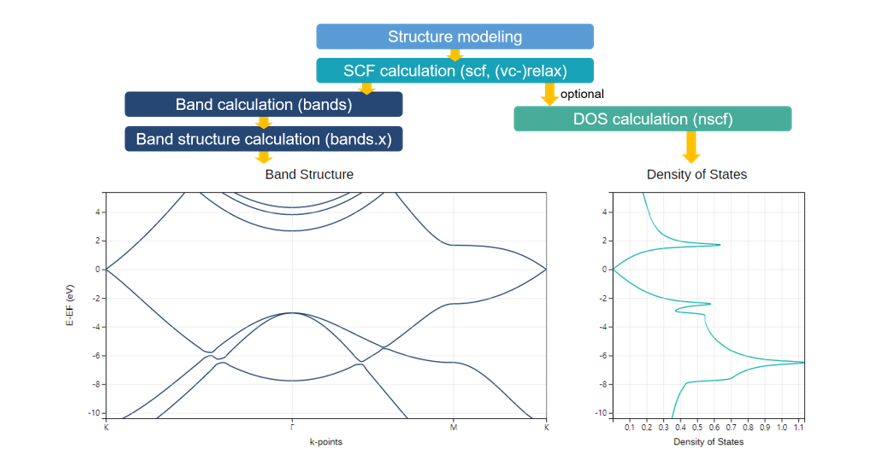To accurately draw a band structure, you need to set a k-point sampling path by passing the high symmetry point of the Brillouin zone, which depends on the crystal system. If a k-point is set to automatic, as k-points are uniformly sampled in a space, the shape of the band structure is not smooth. However, if a k-point path is set to a high symmetric point, then the shape is smooth.

##### 21. Electric Field
2019-09-05 06:05:11Using the Electric field correction option, we can adjust the bandgap of Graphene bilayer by applying the electric field to the Graphene bilayer.

##### 23. Spin-orbit Coupling
2019-09-18 09:14:22According to the relativistic theory, the dipole moments of electrons weakly interact with the orbital motions they make. This interaction is called Spin-orbit coupling (SOC). Applying SOC corrections to the simulation yielding the fine-structure of the system.

Close